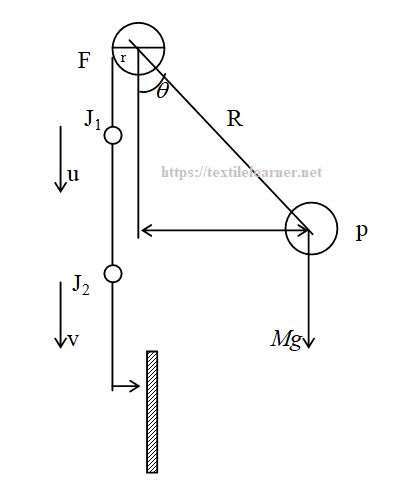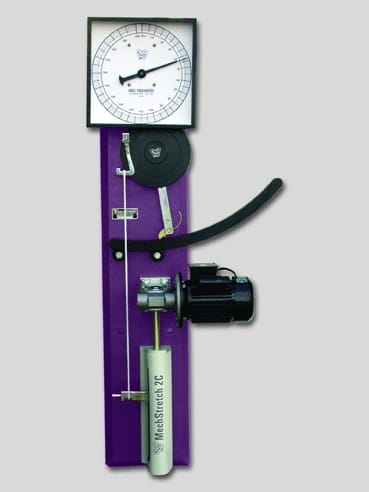# Determination of Cotton Yarn Strength and C.S.P. by Lea Strength Tester

Last Updated on 07/04/2021

Name of the Experiment:
Determination of cotton yarn strength and C.S.P. by lea strength tester.

Theory:
C.S.P is the product of English count and strength of yarn in pound.

i.e. C.S.P = Strength of yarn in pound x Count in English system.

Again, English count is the no. of hanks in 840 yards length per 1 pound weight of yarn.

…………………..L x w
i.e. Count = ————————
…………………..l x W

Here,
L = length of the sample & l = unit length of the system
W = wt. Of the sample & w = unit wt. of the system.Figure: The pendulum lever principle

A lea strength tester measures the strength of one lea yarn. One lea means 120 yards. Strength is a measure of the steady force necessary to break a material and is measured in pound. The machine works in constant rate of extension. Assuming the specimen to be extensible and an absence of any dynamic effects, we get from the figure:

Fr = Mgr = MgRsinq

As the value of MgR and r are constant, therefore,

F ∝ sinθ.

According to the applied force the machine dial gives us the strength in lb on the basis of this q.

Apparatus:

1. Cotton yarn
2. Wrap reel
3. Lea strength tester
4. Electrical balanceFig: Lea strength tester

Machine specification:

• The Lea Strength Tester
• Goodbrand & Co. Ltd.
• Capacity: 100lb

Testing atmosphere:

• Temperature – 29oC and relative humidity – 76%
• Standard atmosphere: temperature – 20oC and relative humidity –  65%.

Sample:

• Size – One lea cotton yarn (1 lea =120 yards).
• No. of sample – 20.

Working procedure:

1. At first one lea cotton yarn is measured by wrap reel and in this way 20 samples are taken for testing.
2. Now, the first sample is fixed with the upper jaw J1 and the lower jaw J2.
3. The m/c is started and observed the dial until the sample is torn out.
4. When the sample is torn out the machine is stopped and the reading is taken.
5. By this way the others’ reading are taken.
6. Then all the samples are weighted and counts are calculated.
7. C.S.P of the all samples are calculated.
8. At last average and CV% are calculated.

Data:

 Reading Yarn length (yds) Yarn strength (lbs) Sample Wt (gms) Yarn Count (Ne) C.S.P of the given sample Avg C.S.P SD% CV% 1 120 86 2.6 24.9 2141.39 2397.16 99.9 4.16 2 120 93 2.63 24.64 2291.52 3 120 97.5 2.7 24 2340 4 120 95 2.62 24.73 2349.34 5 120 98.99 2.60 24.92 2466.83

Calculation:

Suppose for first sample, we find length = 120 yards and weight = 2.315gm.

Now we know, 1 lb = 453.6 gm.

……………………..120 x 1 x 453.6
Then Count = ————————————–
………………………….840 x 2.6

= 24.9 ≈ 25 Ne

And C.S.P = 24.9 x 86 = 2141.39

In this way others are calculated.

Here CV% = 4.16%

Result:

1. The strength of cotton yarn is 97 lb,
2. C.S.P is 2397.16 and
3. The C.V% for C.S.P is 4.16%

Remark:
It is considered that fibers having C.S.P less than 1800 are bad or weak, between 1800-2200 are average and greater than 2200 are strong. From our experiment we see that our samples’ average C.S.P is 2397.16 which is greater than the std 2200 and CV% is 4.16. This is proved that there are many variations in this range but our supplied yarn has good strength. So finally we can say that these yarns have good strength fibers. But it may be vary because our testing atmosphere is not standard.

You may also like:

Share this Article!